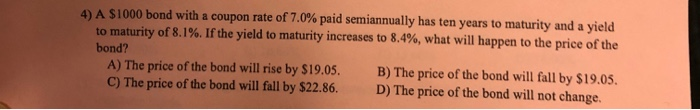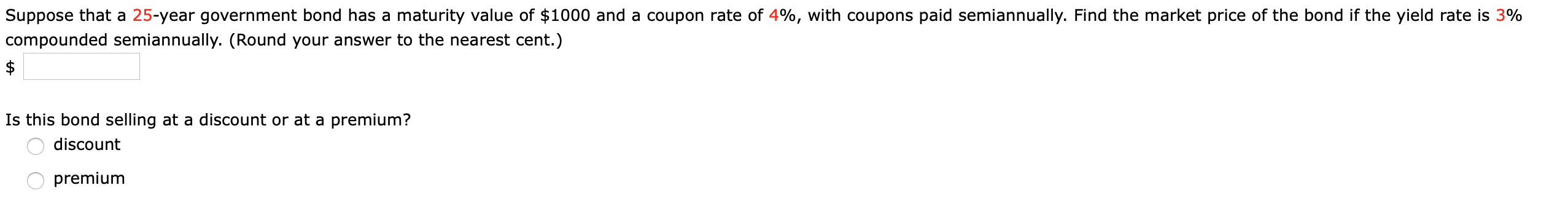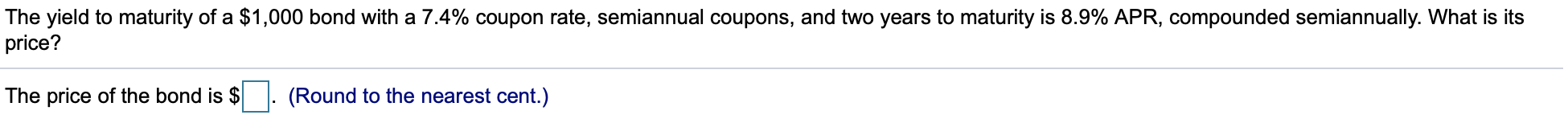Question

What is the coupon rate of a 8-year, \$1000 bond with coupons paid semiannually and a price of \$6900, if it has a yield to maturity of 8.5%.?

Face Value = \$1,000
Current Price = \$6,900

Annual YTM = 8.50%
Semiannual YTM = 8.50% / 2
Semiannual YTM = 4.25%

Time to Maturity = 8 years
Semiannual Period = 16

Let semiannual coupon be \$C

\$6,900 = \$C * PVIFA(4.25%, 16) + \$1,000 * PVIF(4.25%, 16)
\$6,900 = \$C * (1 - (1/1.0425)^16) / 0.0425 + \$1,000 * (1/1.0425)^16
\$6,900 = \$C * 11.440309 + \$513.786847
\$C * 11.440309 = \$6,386.213153
\$C = \$558.22

Semiannual Coupon = \$558.22

Annual Coupon = 2 * Semiannual Coupon
Annual Coupon = 2 * \$558.22
Annual Coupon = \$1,116.44

Coupon Rate = Annual Coupon / Face Value
Coupon Rate = \$1,116.44 / \$1,000
Coupon Rate = 1.1164 or 111.64%

#### Earn Coins

Coins can be redeemed for fabulous gifts.

Similar Homework Help Questions
• ### What is the coupon rate of a 8-year, \$1000 bond with coupons paid semiannually and a...

What is the coupon rate of a 8-year, \$1000 bond with coupons paid semiannually and a price of 6900, if it has a yield to maturity of 8.5%. what is the coupon payment of a 4-year \$1000 bond, 7.5%YTM, and with a 4% coupon rate and quarterly payments subject: finance

• ### What is the coupon rate of a 8-year, \$10000 bond with coupons paid semiannually and a...

What is the coupon rate of a 8-year, \$10000 bond with coupons paid semiannually and a price of \$8900, if it has a yield to maturity of 6%?

• ### Calculate the duration for a 2-year bond which has a 8% annual coupon rate, and coupons...

Calculate the duration for a 2-year bond which has a 8% annual coupon rate, and coupons are paid semiannually. The yield to maturity is 6% and the face value of the bond is \$1000.

• ### Calculate the duration for a 2-year bond which has a 8% annual coupon rate, and coupons...

Calculate the duration for a 2-year bond which has a 8% annual coupon rate, and coupons are paid semiannually. The yield to maturity is 6% and the face value of the bond is \$1000.

• ### A \$ 1000 bond with a coupon rate of 6​% paid semiannually has eight years to...

A \$ 1000 bond with a coupon rate of 6​% paid semiannually has eight years to maturity and a yield to maturity of 9​%. If interest rates rise and the yield to maturity increases to 9.3​%, what will happen to the price of the​ bond?

• ### The yield to maturity of a \$1000 bond with a 6.7% coupon rate, semiannual coupons, and...

The yield to maturity of a \$1000 bond with a 6.7% coupon rate, semiannual coupons, and two years to maturity is 7.6% APR, compounded semiannually, what is the price?

• ### 4) A \$1000 bond with a coupon rate of 7.0 % paid semiannually has ten years...4) A \$1000 bond with a coupon rate of 7.0 % paid semiannually has ten years to maturity and a yield to maturity of 8.1%. If the yield to maturity increases to 8.4%, what will happen to the price of the bond? A) The price of the bond will rise by \$19.05. C) The price of the bond will fall by \$22.86. B) The price of the bond will fall by \$19.05. D) The price of the bond will not...

• ### Suppose that a 25-year government bond has a maturity value of \$1000 and a coupon rate...Suppose that a 25-year government bond has a maturity value of \$1000 and a coupon rate of 4%, with coupons paid semiannually. Find the market price of the bond if the yield rate is 3% compounded semiannually. (Round your answer to the nearest cent.) Is this bond selling at a discount or at a premium? O discount premium

• ### The yield to maturity of a \$1,000 bond with a 7.4% coupon rate, semiannual coupons, and...The yield to maturity of a \$1,000 bond with a 7.4% coupon rate, semiannual coupons, and two years to maturity is 8.9% APR, compounded semiannually. What is its price? The price of the bond is \$ . (Round to the nearest cent.) Suppose a five-year, \$1,000 bond with annual coupons has a price of \$901.23 and a yield to maturity of 5.9%. What is the bond's coupon rate? The bond's coupon rate is %. (Round to three decimal places.)

• ### Suppose a ten-year, \$ 1000 bond with an 8.6 % coupon rate and semiannual coupons is...

Suppose a ten-year, \$ 1000 bond with an 8.6 % coupon rate and semiannual coupons is trading for \$1,035.22. a. What is the bond's yield to maturity (expressed as an APR with semiannual compounding)? b. If the bond's yield to maturity changes to 9.3 %APR, what will be the bond's price?